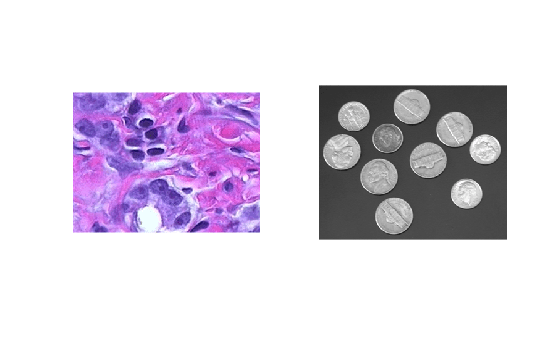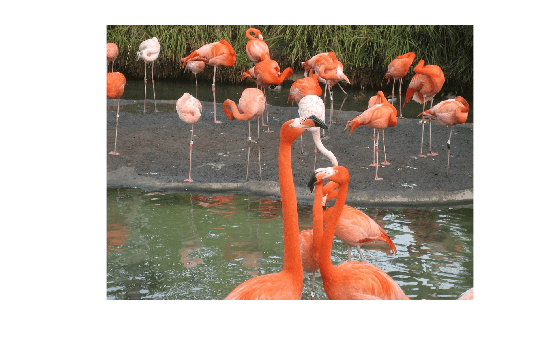Main Content

# imagemodel

Image model object

## Description

An image model object stores information about an image such as class, type, display range, width, height, minimum intensity value and maximum intensity value.

The image model object supports functions that you can use to access this information, get information about the pixels in an image, and perform special text formatting. An `imagemodel` object works by querying the target image `CData`.

## Creation

### Syntax

``imgmodel = imagemodel(himage)``

### Description

example

````imgmodel = imagemodel(himage)` creates an image model object associated with a target image `himage`.If `himage` is an array of image objects, then `imgmodel` is an array of image model objects.```

### Input Arguments

expand all

Target image, specified as a handle or array of handles to image objects.

## Object Functions

 `getClassType` Get class of image from image model `getDisplayRange` Get display range of image from image model `getImageHeight` Get height of image from image model `getImageType` Get type of image from image model `getImageWidth` Get width of image from image model `getMaxIntensity` Get maximum value of image from image model `getMinIntensity` Get minimum value of image from image model `getNumberFormatFcn` Get function handle that converts numeric value into character vector `getPixelInfoString` Get pixel value as character vector `getPixelRegionFormatFcn` Get function handle that formats pixel value into character vector `getPixelValue` Get pixel value as numeric array `getDefaultPixelInfoString` Get default pixel value as character vector `getDefaultPixelRegionString` Get type of information displayed in Pixel Region tool as character vector `getScreenPixelRGBValue` Get screen value of specified pixel in image model `getimagemodel` Image model object from image object

## Examples

collapse all

Create an image model associated with a single image object.

`h = imshow('peppers.png');``im = imagemodel(h)`
``` im = IMAGEMODEL object accessing an image with these properties: ClassType: 'uint8' DisplayRange: [] ImageHeight: 384 ImageType: 'truecolor' ImageWidth: 512 MinIntensity: [] MaxIntensity: [] ```

Create an image model for an array of image object handles.

```figure subplot(1,2,1) h1 = imshow('hestain.png'); subplot(1,2,2) h2 = imshow('coins.png');````im = imagemodel([h1 h2])`
``` im = 1x2 array of IMAGEMODEL objects. ```

Pixel values obtained from an `imagemodel` object can be returned in several formats suitable for display in different interactive image processing tools.

Create an image model associated with a color image.

`h = imshow('flamingos.jpg');``im = imagemodel(h)`
``` im = IMAGEMODEL object accessing an image with these properties: ClassType: 'uint8' DisplayRange: [] ImageHeight: 972 ImageType: 'truecolor' ImageWidth: 1296 MinIntensity: [] MaxIntensity: [] ```

Select a pixel by specifying row and column coordinates. This pixel has (row, column) coordinates (100, 200).

```r = 100; c = 200;```

Get the numeric value of the pixel using the `getPixelValue` function.

`pxValue = getPixelValue(im,r,c)`
```pxValue = 1x3 uint8 row vector 104 95 54 ```

Get the default pixel information string using the `getDefaultPixelInfoString` function. This string depends on the type of image but does not use the pixel values. The pixel information string is suitable for use with the Pixel Information tool.

`defaultPxInfoStr = getDefaultPixelInfoString(im)`
```defaultPxInfoStr = '[R G B]' ```

Using the same string format, get the pixel information string for the specified pixel by using the `getPixelInfoString` function.

`pxInfoStr = getPixelInfoString(im,r,c)`
```pxInfoStr = '[104 95 54]' ```

Get the default pixel region string using the `getDefaultPixelRegionString` function. This string depends on the type of image but does not use the pixel values. The pixel region string is suitable for use with the Pixel Region tool.

`defaultPxRegStr = getDefaultPixelRegionString(im)`
```defaultPxRegStr = 'R:000 G:000 B:000' ```

There are two steps to get the pixel region string for the specified pixel in the same string format. First, get a function `formatFcn` that formats numeric pixel values by using the `getPixelRegionFormatFcn` function. Then, specify the row and column coordinate of the pixel as input arguments to `formatFcn` to get the formatted string.

```formatFcn = getPixelRegionFormatFcn(im); pxRegStr = formatFcn(r,c)```
```pxRegStr = 1x1 cell array {'R:104...'} ```

## See Also

Introduced before R2006a

Download ebook